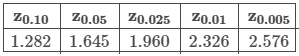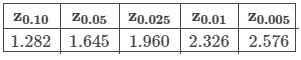Question

Suppose the scores of a standardized test are normally distributed. If the population standard deviation is 4 points, what minimum sample size is needed to be 95% confident that the sample mean is within 1 point of the true population mean?Use the table above for the z-score, and be sure to round up to the nearest integer.Ans: 62QuestionSuppose the weights, in pounds, of the dogs in a city are normally distributed. If the population standard deviation is 3 pounds, what minimum sample size is needed to be 95% confident that the sample mean is within 1 pound of the true population mean?Use the table above for the z-score, and be sure to round up to the nearest integer.Ans: ____________

….Kindly click the purchase icon to purchase the full solution at \$10